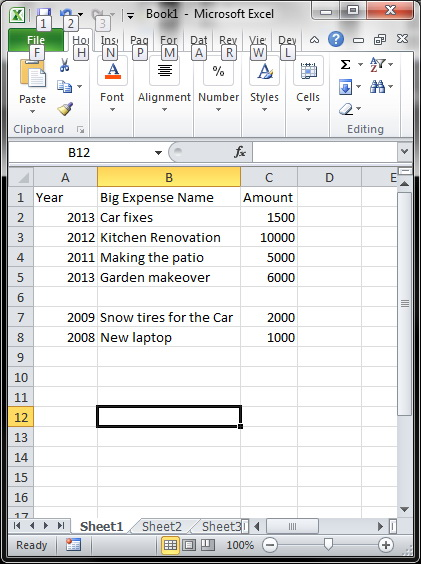# Using VBA macro for Excel

Let’s start with something simple, assume that we have a big spread sheet and we want to highlight it based on a certain subject. For example expenses about car. For the sake of the example we’ll keep it short, but in real life you could be dealing with hundreds of rows.We can see that cell B2 and B7 contains the word “car”, so how you do you highlight these cells using an excel formula? You can’t! The only way to do this is with a VBA macro. VBA macro is much more robust than excel formulas – in essence VBA is a programming for Excel.

So let’s start programming!

1. Enable the VBA development environment. In your Excel, press Alt + F11 . If that doesn’t work:

• Go to File > Options > Customize > Ribbon
• In the main tabs. Go to “Customize The Ribbon” > check “Developer”
• Click on the “Visual Basic” icon.
• A new window will open saying “Microsoft Visual Basic for Applications”

Double click on “Sheet 1”. Then at the top menu go to “Insert” > “Procedure”. Give it a meaningful name “HighlightCarExpenses”

``` Public Sub HighlightCarExpenses() Rem this is a comment Dim emptyCounter, currentRow As Integer Dim currentCell As Range Dim currentCellValue As String```

``` Rem Set initial value for the empty cell counter emptyCounter = 0 currentRow = 1 currentCellValue = "" ```

``` Rem Scan al column B values Rem Stop scanning if we see 3 empty cell in column B Do While (emptyCounter < 3) currentCellValue = ActiveSheet.Cells(currentRow, 2).Value Debug.Print "Value at row " & currentRow & ": " & currentCellValue If (currentCellValue = "") Then emptyCounter = emptyCounter + 1 ElseIf InStr(1, currentCellValue, "car", 1) Then Debug.Print "Car is found at row: " & currentRow ActiveSheet.Cells(currentRow, 2).Interior.Color = RGB(256, 0, 0) End If currentRow = currentRow + 1 Loop Debug.Print "last row" & currentRow End Sub ```

## Glossary

Comment: Part of the code (sentences) that will not be executed by the system. This can be a way for the user/programmer to put a note to her/himself
Debug: Trace a value(s) to find and remove mistake, or just to ensure program correctness.
Dim: A declaration for variable
Integer: A data type that is numerical. Example: 1, 2, 3, etc
String: A data type that is alpha-numerical. Example: "I have 2 dogs", "Expenses are bad", etc. Be aware that a string is always written within double quotes (")